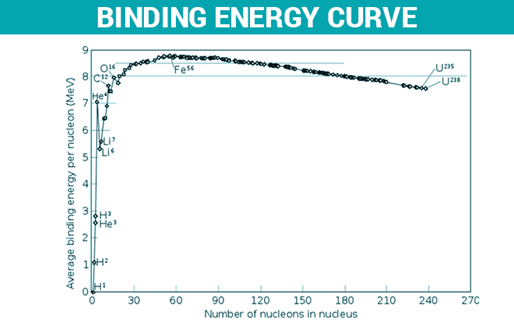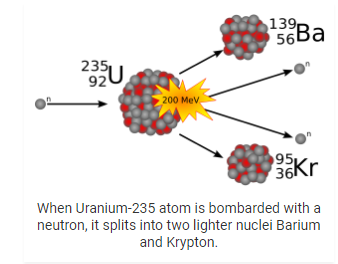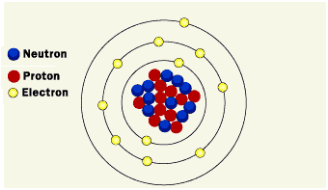# RBSE Solutions For Class 12 Physics Chapter 15: Nuclear Physics | Textbook Important Questions & Answers

The answers are provided for all the questions of Chapter 15 Physics of RBSE Class 12. Students can go through these questions to understand the concepts better and score well in the board examination and entrance examinations for various professional courses. These solutions are provided by the team of experts to provide the best and accurate solutions to the questions. Do check BYJU’S RBSE Class 12 solutions page to get the answers for textbook questions of all subjects.

## Multiple Choice Questions

1. 1.2
2. 2.4
3. 4.8
4. 3.7

Q2: If the mass of 37Li isotope is 7.016005 u and masses of H atom and neutron are respectively 1.007825 u and 1.008665 u then the binding of Li nucleus is

1. 5.6 MeV
2. 8.8 MeV
3. 0.42 MeV
4. 39.2 MeV

Q3: If at some given instant there are 1.024 x 1034 active atoms in some radioactive sample, then number remaining after eight half-lives will be

1. 1.024 x 1020
2. 4.0 x 1017
3. 6.4 x 1018
4. 1.28 x 1019

Q4: For deuteron, the binding energy per nucleon is 1.115 MeV then for this nucleus the mass defect is

1. 2.23 u
2. 0.0024 u
3. 0.0027 u

Q5: In an average life

1. Half of the nuclei decay
2. More than half of the nuclei decay
3. Less than half of the nuclei decay
4. All the nuclei decay

## Very Short Questions

Q1: What is the number of protons and neutrons in the 15X22 nucleus?

Z = 15 (number of protons)

A = 22

A – Z = 22 – 15 = 7(number of neutrons)

Q2: Write energy equivalent (in MeV) of 1μ mass.

Answer: The energy equivalent of 1μ mass is 931.5 MeV.

Q3: A nucleus after β decay converts into its isotope or isobar?

Answer: β decay is radioactive decay in which the nucleus emits beta particles and the original nuclide becomes an isobar.

Q4: For which, α or β rays the energy spectrum is discrete?

Answer: Energy spectrum is discrete for α particles.

Q5: On what type of chain reaction is the working of a nuclear reactor based?

Answer: The nuclear reactor is based on a controlled chain reaction.

Q6: Write the name of any one material used as a moderator in nuclear reactors.

Answer: Graphite is used as a moderator in nuclear reactors.

Q7: Write relation between half-life (T) and decay constant (λ) for a radioactive substance.

T = 2.303 log10 2/λ

T = 0.6932/λ

Q8: What is the SI unit for activity?

Answer: The SI unit for activity is

1 Becquerel = 1 disintegration/second

Q9: After four half-lives how much percentage of a radioactive substance returns?

Q10: Which nuclear reaction is responsible for energy generation in the sun?

Q11: A radioactive element having a mass number 218 and atomic number 84 emits β particles. What are the mass number and atomic number after decay?

Answer: 85X21886X218 + -1e0 + ⊽

The atomic number of the produced nucleus is 85

The mass number is 218

Q12: Is there a loss in mass number after gamma decay?

Answer: After gamma decay, there is no change in mass number of atomic number.

Q13: From which metal is it easier to remove a nucleon, iron or lead?

Answer: Binding energy per nucleon is less for lead than iron. Therefore nucleons can be removed easily from lead.

Q14: A nucleus undergoes fission into two unequal parts. Which of the two parts (heavier or lighter) will have more kinetic energy?

Kinetic energy = p2/2m

Momentum p remains conserved

Kinetic energy ∝ 1/m

Therefore Kinetic energy will be more for the lighter nucleus.

Q15: If the nucleons of a nucleus are well separated from each other total mass increases. From where does this mass come?

Answer: The extra mass comes from the binding energy of the nucleus.

Q1: A hydrogen molecule has two protons and two electrons. In discussing the behaviour of the hydrogen molecule the nuclear force between these protons is always ignored. Why?

Answer: The separation between the protons in the hydrogen molecule is ∼ 70 pm which is much greater than the nuclear force between the protons. Therefore, the nuclear force between protons is always ignored.

Q2: A student claims that a heavier form of hydrogen decays by α emission. What will be your reaction?

Answer: In α decay, the daughter nucleus will have an atomic number less than 2. In the heavier form of hydrogen, this is not possible.

Q3: Define the unified atomic mass unit (u).

Answer: The dalton (Da) or unified atomic mass (u) is defined as one-twelfth of the mass of an unbound carbon-12 atom at rest and in its ground state.

1u = (Mass of one 6C12)/12

= 1.992647 x 10 -26/12

= 1.66054 x 10 -27 kg

Q4: Explain the meaning of nuclear mass defect.

Answer: The sum of individual masses of neutrons and protons is greater than the actual mass of the nucleus in every situation because energy is ejected when the nucleus is created. This difference in the total mass of the original components and the actual mass is called the mass defect. This is the mass that is missing in the final nucleus

Mass defect = mass of the nucleus calculated – Actual mass of the nucleus

Mass defect = (mass of the proton + mass of neutron) – Actual mass of the nucleus

Answer: Radioactivity is the nuclear phenomenon where heavy mass elements like uranium, thorium, radium etc will decay spontaneously emitting α,β or у radiations. This process does not depend on external parameters like pressure, temperature, Phase change and chemical combinations.

Q6: Mention Rutherford – Soddy decay law.

Answer: According to Rutherford – Soddy decay law, the rate of radioactive decay at any instant is proportional to the number of active nuclei present at that instant.

– dN/dt = λN, here λ is the decay constant

The number of active nuclei N at time t is given by

N = N0e-λt

N0 is the active nuclei at time t=0

The number of active nuclei decreases exponentially with time

Q7: Give definitions of half-life and mean-life of a radioactive substance and write relation between them.

Half-life is the time interval in which the number of active nuclei in a radioactive element reduces to half of its initial value N0. If the half-life of a radioactive element is denoted as T. The equation N = N0e-λt is written as N0/2 = N0e-λT (t = T)

e-λT = 2

T = loge 2/λ

T = 2.303 log10 2/λ

T = 0.6932/λ

Mean-life or average life of a radioactive substance is the ratio of the total lifetime of all the radioactive atoms to the total number of such atoms in it.

Q8: What is α decay? What is the type of α particle energy spectrum?

Answer: In an α decay a heavy mass nucleus (X) known as the parent nucleus changes into another nucleus by emitting α particles.

ZXAZ-2 YA-4 + 2He4

The atomic number of Y is 2 less than X. The mass number decreases by 4.

α particle energy spectrum is a set of discrete energies suggesting quantization of nuclear energy levels.

Q9: β ray energy spectrum is continuous? What is the meaning of this?

Answer: The disintegration energy Q appears in the form of the kinetic energy of decay products. Because of the higher mass of the residual nucleus, it can be assumed that the energy is shared in varying proportions between the electron and neutrino (antineutrino). The sum of the energy of the electron and neutrino (antineutrino) will be equal to Q. Therefore, the emitted electron will have any value between zero and Q.

Q10: The neutrino hypothesis is helpful in describing which conservation law is in the β decay process?

Answer: The rest mass of the neutrino is zero but it has a momentum due to energy. The momentum conservation can be explained by considering the vector sum of the momentum of electron and neutrino must be equal and opposite to the recoiling residual nucleus. The neutrino is given a spin ½ to hold the conservation of angular momentum in β decay.

Q11: Write any two properties of the nuclear forces?

1. Nuclear forces are independent of electric charges
2. The range of the nuclear force is very short

Q12: What do you mean by binding energy per nucleon? How is it related to nuclear stability?

Answer: The binding energy per nucleon (ΔEb) is determined by dividing the binding energy(Eb) by the mass number (A). A higher value of binding energy per nucleon indicates more stability of the nucleus.A graph plotted between binding energy per nucleon and number of nucleons shows that middle mass nuclei are more stable than light mass or heavy mass nuclei.

Q13: Define nuclear fission.

Answer: A high mass nucleus breaks into two middle mass nuclei through a nuclear reaction called nuclear fission. This process can be caused by radioactive decay naturally or can be simulated by achieving necessary conditions.The resulting elements have a combined mass which is less than the actual element. The difference in their mass gets converted into nuclear energyQ14: What is meant by critical mass in reference to a nuclear chain reaction.

Answer: The multiplication factor determines if the mass of the fissionable material can sustain a chain reaction or not. The size of the material for which multiplication factor k =1 is called critical size and the mass corresponding to the critical size is called critical mass. The chain reaction remains sustained.

Q15: Heavy water is a good moderator in nuclear reactors? Why?

Answer: Moderator is required to slow down the neutron in a nuclear fission reaction so that chain reaction is steady or sustained. Heavy water has a high moderating ratio. Therefore, it is a good moderator in nuclear reactors .

## Essay Type Questions

Q1: Describe composition of nucleus and discuss nuclear forces?

Composition of Nucleus

The Nucleus of an atom consists of a tightly packed arrangement of protons and neutrons. These are the two heavy particles in an atom and hence 99.9% of the mass is concentrated in the nucleus. Of the two, the protons possess a net positive charge and hence the nucleus of an atom is positively charged on the whole and the negatively charged electrons revolve around the central nucleus. Since the mass concentration at the nucleus of an atom is immense the nuclear forces holding the protons and the neutrons together are also large. The protons are in such close vicinity to each other inside the tiny nucleus and therefore the electrostatic forces of repulsion also act inside the nucleus. The total number of protons in a nucleus is equal to the number of electrons revolving around the nucleus and hence the atom, on the whole, is electrically neutral.Nuclear Forces

The force that binds the protons and neutrons together is called nuclear force. The nuclear force is stronger than the repulsive Coulomb force between the protons and thus the nucleus stays bound.

• Nuclear forces do not depend on the charge of the elements. For a given separation the nuclear force is the same between proton-proton, neutron- neutron or proton-neutron. Electrons are not affected by nuclear nuclear forces.
• Nuclear forces are short-range forces. The range up to which the nuclear force acts is called nuclear range and it is of the order of a few femtometer.
• Nuclear forces are non central in nature. The force between the nucleons also depends on the orientation of spins of the nucleons.
• Nuclear forces are attractive in nature. However, if the separation is less than 1fm the nuclear force tends to be repulsive.

Q2: What is meant by nuclear fission? Why is a nuclear fission chain not self-sustained?

Explain what is to be done to make a chain reaction sustained.

Answer: A high mass nucleus breaks into two middle mass nuclei through a nuclear reaction called nuclear fission. This process can be caused by radioactive decay naturally or can be simulated by achieving necessary conditions. The resulting elements have a combined mass which is less than the actual element. The difference in their mass gets converted into nuclear energyThe nuclear fission reaction is not self sustained because of

1. The neutron leakage problem
2. The neutron energy problem
3. The neutron capture problem

The chain reaction can be sustained by doing the following things

1. The neutron leakage problem: A certain percentage of the neutrons obtained will leak out the fissionable material. Some of the neutrons are absorbed by the shields enclosing the fissionable materials. Leakage is a surface affect.The fraction of the material leakage can be reduced by making the material volume large enough so that there is a decrease in surface to volume ratio. There is a minimum size or minimum mass of the fissionable material for the chain reaction to continue is called critical mass.
2. The neutron energy problem: Nuclear fission takes place due to slow neutrons. Therefore, the fast neutrons are slowed down using moderators.
3. The neutron capture problem: The 238U used as fuel absorbs the neutrons. To avoid this moderators and 238U rods are arranged in such a way that the neutrons come in contact with the 238U rods only after moderation